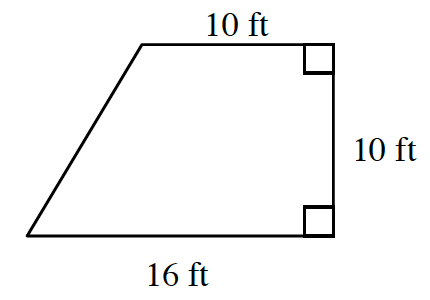### Home > CC2MN > Chapter 4 > Lesson 4.3.1 > Problem4-78

4-78.

Dante is ordering wood flooring for his bedroom, which is shaped like a trapezoid (shown at right). If the flooring materials cost $\5$ per square foot, how much should he expect to pay for the materials?

First, you must find the area of the trapezoid, since this in the amount of flooring material Dante will need.

$\frac{1}{2} (10)(16 + 10) = 5(26) = 130$ square feet

Next, multiply the area by $\5$ to find the cost of the necessary flooring materials. $(130)(5)=\650$

$\650$